Fill void regions in 2-D and split cells in 3-D geometry

Since R2020a

## Syntax

``h = addFace(g,edges)``
``[h,FaceID] = addFace(g,edges)``

## Description

example

````h = addFace(g,edges)` adds a new face to the geometry `g`. The specified edges must form a closed contour. For a 2-D geometry, adding a new face lets you fill voids in the geometry. For a 3-D geometry, adding a new face lets you split one cell into multiple cells.You can add several new faces simultaneously by specifying their contours in a cell array. Each contour in the cell array must be unique. NoteAfter modifying a geometry, always call `generateMesh` to ensure a proper mesh association with the new geometry. ```

example

````[h,FaceID] = addFace(g,edges)` also returns a row vector containing IDs of the added faces.```

## Examples

collapse all

Add a face to a 2-D geometry to fill an internal void.

Create a PDE model.

`model = createpde();`

Import the geometry. This geometry has one face.

`gm = importGeometry(model,"PlateSquareHolePlanar.stl")`
```gm = DiscreteGeometry with properties: NumCells: 0 NumFaces: 1 NumEdges: 8 NumVertices: 8 Vertices: [8x3 double] ```

Plot the geometry and display the face labels.

`pdegplot(gm,"FaceLabels","on")`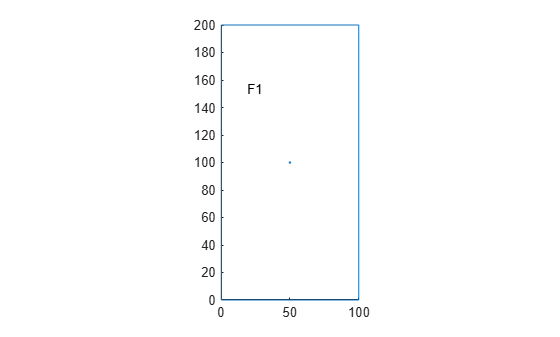Zoom in and display the edge labels of the small hole at the center.

```figure pdegplot(gm,"EdgeLabels","on") axis([49 51 99 101])```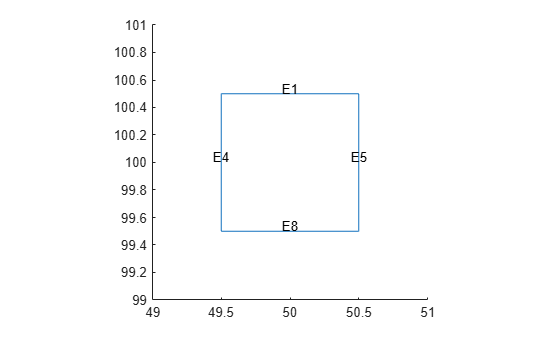Fill the hole by adding a face. The number of faces in the geometry changes to 2.

`gm = addFace(gm,[1 8 4 5])`
```gm = DiscreteGeometry with properties: NumCells: 0 NumFaces: 2 NumEdges: 8 NumVertices: 8 Vertices: [8x3 double] ```

Plot the modified geometry and display the face labels.

`pdegplot(gm,"FaceLabels","on")`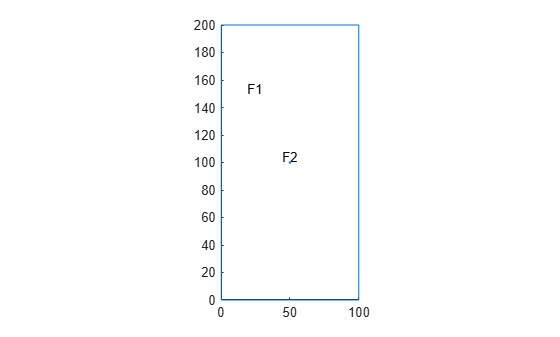Add a face in a 3-D geometry to split a cell into two cells.

Create a PDE model.

`model = createpde();`

Import the geometry. The geometry consists of one cell.

`gm = importGeometry(model,"MotherboardFragment1.stl")`
```gm = DiscreteGeometry with properties: NumCells: 1 NumFaces: 26 NumEdges: 46 NumVertices: 34 Vertices: [34x3 double] ```

Plot the geometry and display the edge labels. Zoom in on the corresponding part of the geometry to see the edge labels there more clearly.

```pdegplot(gm,"EdgeLabels","on","FaceAlpha",0.5) xlim([-0.05 0.05]) ylim([-0.05 0.05]) zlim([0 0.05])```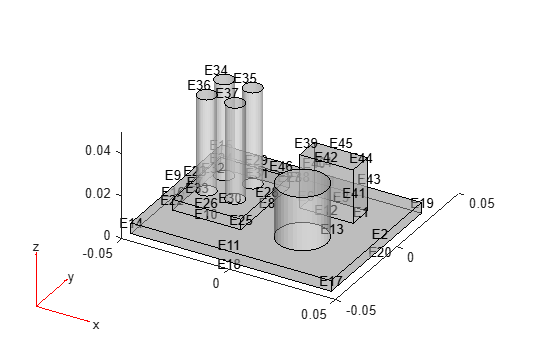Split the cuboid on the right side into a separate cell. For this, add a face bounded by edges 1, 3, 6, and 12.

`[gm,ID] = addFace(gm,[1 3 6 12])`
```gm = DiscreteGeometry with properties: NumCells: 2 NumFaces: 27 NumEdges: 46 NumVertices: 34 Vertices: [34x3 double] ```
```ID = 27 ```

Plot the modified geometry and display the cell labels.

`pdegplot(gm,"CellLabels","on","FaceAlpha",0.5)`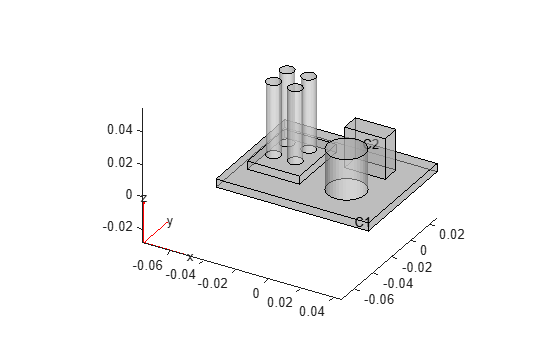Now split the cuboid on the left side of the board and all cylinders into separate cells by adding a face at the bottom of each shape. To see edge labels more clearly, zoom and rotate the plot. Use a cell array to add several new faces simultaneously.

```[gm,IDs] = addFace(gm,{[5 7 8 10], ... 30, ... 31, ... 32, ... 33, ... 13})```
```gm = DiscreteGeometry with properties: NumCells: 8 NumFaces: 33 NumEdges: 46 NumVertices: 34 Vertices: [34x3 double] ```
```IDs = 6×1 28 29 30 31 32 33 ```

Plot the modified geometry and display the cell labels.

`pdegplot(gm,"CellLabels","on","FaceAlpha",0.5)`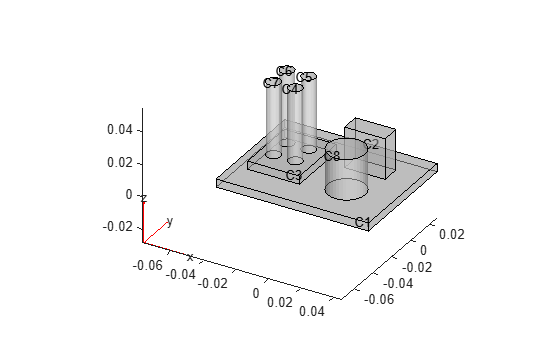## Input Arguments

collapse all

Geometry, specified as an `fegeometry` object, a `DiscreteGeometry`, or an `AnalyticGeometry` object. See `fegeometry`, DiscreteGeometry Properties, and AnalyticGeometry Properties.

Edges forming a unique closed flat contour, specified as a vector of positive integers or a cell array of such vectors. You can specify edges within a vector in any order.

When you use a cell array to add several new faces, each contour in the cell array must be unique.

Example: `addFace(g,[1 3 4 7])`

## Output Arguments

collapse all

• If the original geometry `g` is an `fegeometry` object, then `h` is a new `fegeometry` object representing the modified geometry. The original geometry `g` remains unchanged.

• If the original geometry `g` is a `DiscreteGeometry` `AnalyticGeometry` object, then `h` is a handle to the modified object `g`.

Face ID, returned as a positive number or a row vector of positive numbers. Each number represents a face ID. When you add a new face to a geometry with N faces, the ID of the added face is N + 1.

## Tips

• `addFace` errors when the specified contour defines an already existing face.

• If the original geometry `g` is a `DiscreteGeometry` or `AnalyticGeometry` object, `addFace` modifies the original geometry `g`.

• If the original geometry `g` is an `fegeometry` object, and you want to replace it with the modified geometry, assign the output to the original geometry, for example, `g = addFace(g,[1 3 4 7])`.

## Version History

Introduced in R2020a

expand all